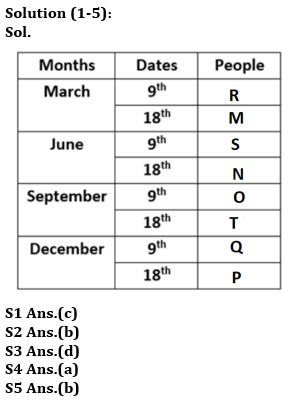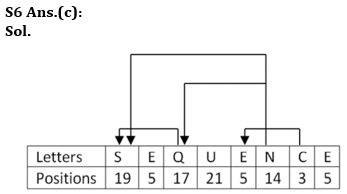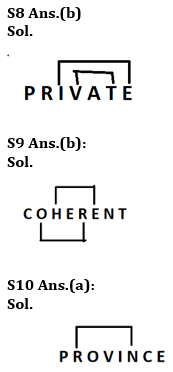Latest Banking jobs   »

# Reasoning Quiz For Bank of Baroda AO 2023-29th April

Directions (1-5): Study the following information carefully and answer the given questions.
Eight people i.e., M, N, O, P, Q, R, S and T were born on two different dates i.e., 9th and 18th of March, June, September, and December of the same year but not necessarily in the same order. M was born in a month having 31 days on an even-numbered date. Three people were born between M and T. Number of people who were born before S is the same as the number of people who were born after T. Number of people born between S and M is the same as the number of people born between S and N. Three people were born between N and P. O was born before T. One person was born between O and Q. R was born before O. O was born in the month which has even number of days.

Q1. How many people were born between O and R?
(a) More than three
(b) One
(c) Three
(d) None
(e) Two

Q2. Four of the following five are alike in a certain way and hence form a group. Who among the following doesn’t belong to that group?
(a) T
(b) R
(c) M
(d) N
(e) P

Q3. Who amongst the following was born in 9th March?
(a) Q
(b) T
(c) S
(d) R
(e) None of these

Q4. How many people were born after T?
(a) Two
(b) One
(c) Three
(d) Four
(e) None of these

Q5. Q was born in which of the following dates?
(a) 18th December
(b) 9th December
(c) 9th June
(d) 9th March
(e) None of these

Q6. How many such pairs of letters are there in the word SEQUENCE each of which has as many letters between them in the word in both forward and backward directions as in the English alphabet?
(a) Three
(b) Two
(c) Four
(d) Five
(e) None

Q7. If it is possible to make a meaningful word, using the third, fourth, seventh and eighth letters of the word ‘APPLICANT’, then what is the fourth letter from the left of the word formed? If no such word be formed, mark the answer as ‘M’. If more than one word can be formed mark the answer as ‘X’.
(a) A
(b) N
(c) P
(d) X
(e) M

Q8. How many such pairs of letters are there in the word PRIVATE each of which has as many letters between them in the word in both forward and backward directions as in the English alphabet?
(a) Three
(b) Two
(c) Four
(d) Five
(e) None

Q9. How many such pairs of letters are there in the word COHERENT each of which has as many letters between them in the word in both forward and backward directions as in the English alphabet?
(a) Three
(b) Two
(c) Four
(d) Five
(e) None

Q10. How many such pairs of letters are there in the word PROVINCE each of which has as many letters between them in the word in both forward and backward directions as in the English alphabet?
(a) One
(b) Two
(c) Four
(d) Five
(e) None

Directions (11-15): In these questions, the relationship between different elements is shown in the statements. These statements are followed by two conclusions.

Q11. Statements: W ≤ S < B, M > S.
Conclusions: I. B ≥ W
II. M < B
(a) If only conclusion I follows.
(b) If only conclusion II follows.
(c) If either conclusion I or II follows.
(d) If neither conclusion I nor II follows.
(e) If both conclusions I and II follow.

Q12. Statements: S ≥ Y, H < D ≤ S, J = Y
Conclusions: I. S > J
II. H < J
(a) If only conclusion I follows.
(b) If only conclusion II follows.
(c) If either conclusion I or II follows.
(d) If neither conclusion I nor II follows.
(e) If both conclusions I and II follow.

Q13. Statements: B > T ≥ R < U, N > R = K
Conclusions: I. B > K
II. T > K
(a) If only conclusion I follows.
(b) If only conclusion II follows.
(c) If either conclusion I or II follows.
(d) If neither conclusion I nor II follows.
(e) If both conclusions I and II follow.

Q14. Statements: M < Z = Y, D ≤ M
Conclusions: I. D ≤ Y
II. D < Z
(a) If only conclusion I follows.
(b) If only conclusion II follows.
(c) If either conclusion I or II follows.
(d) If neither conclusion I nor II follows.
(e) If both conclusions I and II follow.

Q15. Statements: K > S, L ≤ F, S = L
Conclusions: I. K > F
II. S ≤ F
(a) If only conclusion I follows.
(b) If only conclusion II follows.
(c) If either conclusion I or II follows.
(d) If neither conclusion I nor II follows.
(e) If both conclusions I and II follow.

SolutionsS7 Ans.(c):
Sol. PLANSolutions (11-15):
S11 Ans.(d)
Sol.
I. B ≥ W – False
II. M < B – False
So, neither I nor II follows.

S12 Ans.(d)
Sol.
I. S > J – False
II. H < J – False
So, neither I nor II follows.

S13 Ans.(a):
Sol.
I. B > K – True
II. T > K – False.
So, only conclusion I follows.

S14 Ans.(b):
Sol.
I. D ≤ Y – False
II. D < Z – True.
So, only conclusion II follows.

S15 Ans.(b):
Sol.
I. K > F – False
II. S ≤ F – True.
So, only conclusion II follows.## FAQs

### How many sections are there in the IRDAI exam?

There are 4 sections in the IRDAI assistant manager online preliminary exam. They are Reasoning, English Language, General awareness, Quantitative aptitude.

#### Congratulations!Union Budget 2023-24: Free PDF